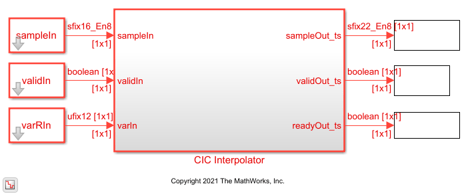# Implement CIC Interpolator Filter for HDL

This example shows how to use the CIC Interpolator block to filter and upsample data. This block supports scalar and vector inputs. To work with scalar and vector inputs separately, this example uses two Simulink® models. You can generate HDL code from the subsystems in these Simulink models.

### Set Up Input Data Parameters

Set up these workspace variables to configure the CIC Interpolator block. This block supports fixed and variable interpolation rates for scalar inputs and only a fixed interpolation rate for vector inputs. The example runs the `HDLCICInterpolatorModel.slx` model when you set the `scalar` value to `true` and runs the `HDLCICInterpolatorModelVectorSupport.slx` model when you set the `scalar` value to `false`. For scalar inputs, choose a range for the input `varRValue` values and set the interpolation factor value, `R`, to the maximum expected interpolation factor. For vector inputs, the input data must be a column vector of size 1 to 32.

```R = 8; % interpolation factor M = 1; % differential delay N = 3; % number of sections scalar = true; % true for scalar; false for vector if scalar varRValue = [2,4,R]; vecSize = 1; modelname = "HDLCICInterpolatorModel"; else varRValue = R; %#ok vecSize = 1; modelname = "HDLCICInterpolatorModelVectorSupport"; end numFrames = length(varRValue); dataSamples = cell(1,numFrames); varRtemp = cell(1,numFrames); cicFcnOutput = []; WL = 0; % word length FL = 0; % fraction length ```

### Generate Reference Output from `dsp.CICInterpolator` System Object

To generate reference output data for comparison, apply the samples to the `dsp.CICInterpolator` System object™. This System object does not support variable interpolation rates, so you must create and release the object for each change in the interpolation factor.

```for i = 1:numFrames framesize = 4; dataSamples{i} = fi(randn(framesize,1),1,16,8); varRtemp{i} = fi(varRValue(i)*ones(framesize,1),0,12,0); obj = dsp.CICInterpolator("DifferentialDelay",M,"NumSections",N, ... "InterpolationFactor",varRValue(i)); cicOut = step(obj,dataSamples{i}).'; WL = max([WL,cicOut.WordLength]); FL = max([FL,cicOut.FractionLength]); cicFcnOutput = [fi(cicFcnOutput,1,WL,FL),cicOut]; release(obj); end ```

### Convert Input to Stream of Samples and Import to Simulink Model

Generate a stream of samples by converting frames to samples. Provide those samples (`sampleIn`) as input to the Simulink model along with the corresponding valid signal (`validIn`) and interpolation rate (`varRIn`). The latency of the block for scalar and vector inputs is calculated based on the type of input and the number of sections, `N`. For more information about latency, see the Gain correction parameter. To flush remaining data before changing the interpolation rate, run the model by inserting the required number of idle cycles after each frame using the `idlecyclesbetweenframes` value.

```idlecyclesbetweensamples = R; % upsampling the inputs by factor R idlecyclesbetweenframes = floor((vecSize-1)*(N/vecSize)) + 1 + N ... + (2+(vecSize+1)*N) + 9; sampleIn = []; validIn = []; varRIn = []; len = 0; for ij = 1:numFrames dataInFrame = reshape([0; dataSamples{ij}],vecSize,[]); len = size(dataInFrame,2); data = []; valid= []; varR = []; for ii = 1:len data = [data dataInFrame(:,ii) ... zeros(vecSize,idlecyclesbetweensamples)]; %#ok valid = [valid true(1,1) ... false(1,idlecyclesbetweensamples)]; %#ok varR = [varR varRtemp{ij}(1) ... zeros(1,idlecyclesbetweensamples)]; %#ok end sampleIn = cast([sampleIn,data, ... zeros(vecSize,idlecyclesbetweenframes)],"like",dataInFrame); validIn = logical([validIn,valid,zeros(1,idlecyclesbetweenframes)]); varRIn = fi([varRIn,varR,zeros(1,idlecyclesbetweenframes)],0,12,0); end sampleIn = sampleIn.'; sampletime = 1; simTime = length(validIn); ```

Run the model. Running the model imports the input signal variables from the MATLAB® workspace to the CIC Interpolator block in the model.

```open_system(modelname); sim(modelname); ```### Compare Simulink Block Output with MATLAB System Object Output

Capture the output of the Simulink block. Compare the captured output with the output of the `dsp.CICInterpolator` System object.

```sampleOut = squeeze(sampleOut_ts.Data).'; validOut = squeeze(validOut_ts.Data); cicOutput = sampleOut(validOut); fprintf('\nCIC Interpolator\n'); difference = (abs(cicOutput-cicFcnOutput(1:length(cicOutput)))>0); fprintf(['\nTotal number of samples differed between Simulink block ' ... 'output and MATLAB System object output is: %d \n'],sum(difference)); ```
```CIC Interpolator Total number of samples differed between Simulink block output and MATLAB System object output is: 0 ```